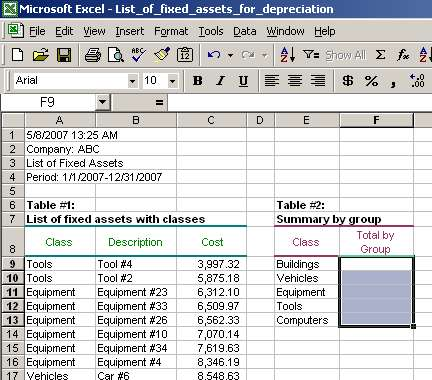# Sumif Function, Sumif Syntax, Sumif Examples

## This Excel lecture explains sumif function in Excel. Two sumif examples are provided to illustrate the power of sumif function. Sumif is very useful in summarizing (accounting) data. You can use a pivot table or sumif function to summatize numbers based on a criteria, and sumif can be more flexible than a pivot table in some situations.

The company name and numbers in this lecture are not real and no association with a real company with a similar name, if such exists, should be made.

### 1) Sumif syntax and arguments

SUMIF function summarizes values in cells specified by a given criteria. The function has three arguments and syntax described below. Note that there are white spaces presented in the below formula line; in some earlier Excel version such white spaces will cause an error message and in some later Excel versions, white spaces are treated without problems. So, if your Excel version gives you an error, just delete all white spaces within the formula:

= SUMIF ( range, criteria, sum_range )

Let's review the function arguments in more detail.

• range is the range of cells you want evaluated. Such cells will be compared to criteria and if the criteria is met, the cell will be included into the formula result;
• criteria is the criteria in the form of a number, expression, or text that defines which cells will be added. For example, criteria can be expressed as 100, "100", ">100", "oranges". Note that the formula compares values in range cells and if the values meet criteria, such cells are included into the formula result;
• sum_range are the actual cells to sum. The cells in sum_range are summed only if their corresponding cells in range match the criteria. If sum_range is omitted, the cells in range are summed.

We will take a look at an example to see how all above is tied together.

### 2) Sumif example #1: Summarize cost of fixed assets by class using SUMIF function

We have a list of fixed assets with fixed asset classes (Table #1, cells A8-D179). We also have a table with fixed asset classes in one column (Table #2, cells E8-G13) and an empty column (Table #2, cells F8-G13) where we would enter our totals. See the list of fixed assets with classes:Column E in Table #2 is a list of unique fixed asset classes (i.e., Buildings, Vehicles, etc.). Column F in Table #2 is where we are going to calculate totals for respective classes (i.e., total of fixed asset costs for Buildings, etc.).

Page 1 of 3

Next